Next: Exercises Up: Planetary Motion Previous: Motion in a General

Motion in a Nearly Circular Orbit

In principle, a circular orbit is a possible orbit for any attractive central force. However, not all forces result in stable circular orbits. Let us now consider the stability of circular orbits in a general central force-field. Equation (250) generalizes to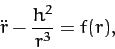(305)

where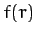is the radial force per unit mass. For a circular orbit,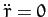, and the above equation reduces to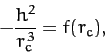(306)

where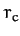is the radius of the orbit.

Let us now consider small departures from circularity. Let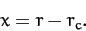(307)

Equation (305) can be written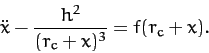(308)

Expanding the two terms involving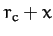as power series in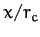, and keeping all terms up to first order, we obtain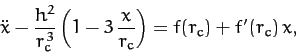(309)

where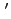denotes a derivative. Making use of Equation (306), the above equation reduces to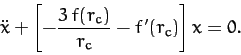(310)

If the term in square brackets is positive then we obtain a simple harmonic equation, which we already know has bounded solutions--i.e., the orbit is stable to small perturbations. On the other hand, if the term is square brackets is negative then we obtain an equation whose solutions grow exponentially in time--i.e., the orbit is unstable to small oscillations. Thus, the stability criterion for a circular orbit of radiusin a central force-field characterized by a radial force (per unit mass) functionis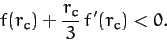(311)

For example, consider an attractive power-law force function of the form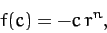(312)

where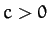. Substituting into the above stability criterion, we obtain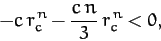(313)

or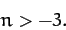(314)

We conclude that circular orbits in attractive central force-fields which decay faster than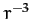are unstable. The case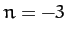is special, since the first-order terms in the expansion of Equation (308) cancel out exactly, and it is necessary to retain the second-order terms. Doing this, it is easily demonstrated that circular orbits are also unstable for inverse-cube () forces.

An apsis is a point in an orbit at which the radial distance,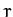, assumes either a maximum or a minimum value. Thus, the perihelion and aphelion points are the apsides of planetary orbits. The angle through which the radius vector rotates in going between two consecutive apsides is called the apsidal angle. Thus, the apsidal angle for elliptical orbits in an inverse-square force-field is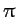.

For the case of stable nearly circular orbits, we have seen thatoscillates sinusoidally about its mean value,. Indeed, it is clear from Equation (310) that the period of the oscillation is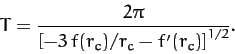(315)

The apsidal angle is the amount by whichincreases in going between a maximum and a minimum of. The time taken to achieve this is clearly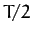. Now,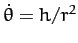, where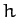is a constant of the motion, andis almost constant. Thus,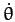is approximately constant. In fact,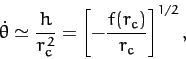(316)

where use has been made of Equation (306). Thus, the apsidal angle,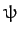, is given by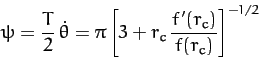(317)

For the case of attractive power-law central forces of the form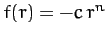, where, the apsidal angle becomes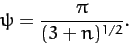(318)

Now, it should be clear that if an orbit is going to close on itself then the apsidal angle needs to be a rational fraction of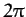. There are, in fact, only two small integer values of the power-law index,, for which this is the case. As we have seen, for an inverse-square force law (i.e.,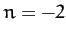), the apsidal angle is. For a linear force law (i.e.,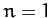), the apsidal angle is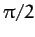--see Section 4.2. However, for quadratic (i.e.,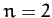) or cubic (i.e.,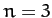) force laws, the apsidal angle is an irrational fraction of, which means that non-circular orbits in such force-fields never close on themselves.

Let us, finally, calculate the apsidal angle for a nearly circular orbit of radiusin a slightly modified (attractive) inverse-square force law of the form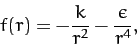(319)

where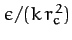is small. Substitution into Equation (317) yields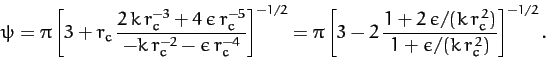(320)

Expanding to first-order in, we obtain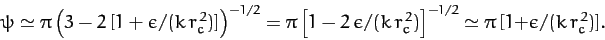(321)

We conclude that if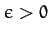then the perihelion (or aphelion) of the orbit advances by an angle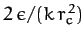every rotation period. It turns out that the general relativistic corrections to Newtonian gravity give rise to a small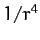modification (with) to the Sun's gravitational field. Hence, these corrections generate a small precession in the perihelion of each planet orbiting the Sun. This effect is particularly large for Mercury--see Section 12.13.Next: Exercises Up: Planetary Motion Previous: Motion in a General
Richard Fitzpatrick 2011-03-31